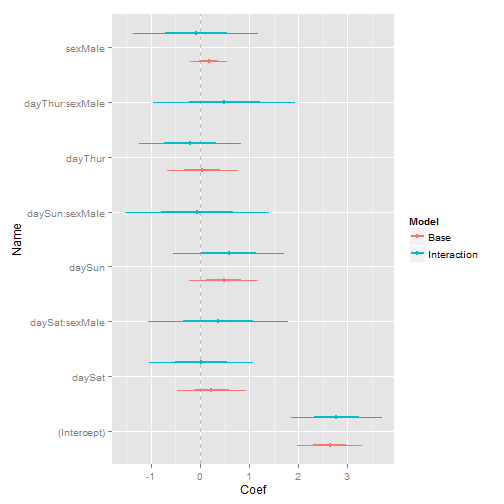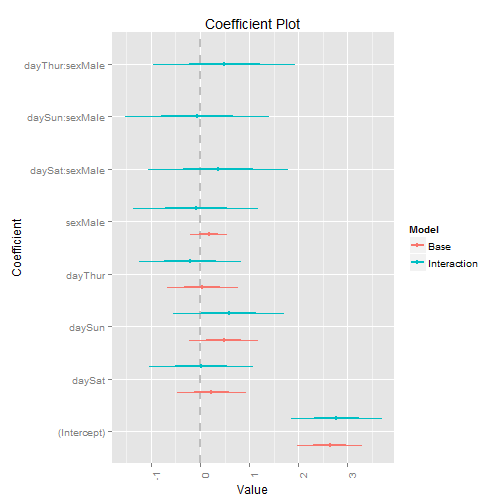An often requested feature for Hadley Wickham's ggplot2 package is the ability to vertically dodge points, lines and bars. There has long been a function to shift geoms to the side when the x-axis is categorical: position_dodge. However, no such function exists for vertical shifts when the y-axis is categorical. Hadley usually responds by saying it should be easy to build, so here is a hacky patch.

All I did was copy the old functions (geom_dodge, collide, pos_dodge and PositionDodge) and make them vertical by swapping y's with x's, height with width and vice versa. It's hacky and not tested but seems to work as I'll show below.

First the new functions:

``````require(proto)
``````
``````## Loading required package: proto
``````
``````collidev <- function(data, height = NULL, name, strategy, check.height = TRUE) {
if (!is.null(height)) {
if (!(all(c("ymin", "ymax") %in% names(data)))) {
data <- within(data, {
ymin <- y - height/2
ymax <- y + height/2
})
}
} else {
if (!(all(c("ymin", "ymax") %in% names(data)))) {
data\$ymin <- data\$y
data\$ymax <- data\$y
}
heights <- unique(with(data, ymax - ymin))
heights <- heights[!is.na(heights)]
if (!zero_range(range(heights))) {
warning(name, " requires constant height: output may be incorrect",
call. = FALSE)
}
height <- heights
}
data <- data[order(data\$ymin), ]
intervals <- as.numeric(t(unique(data[c("ymin", "ymax")])))
intervals <- intervals[!is.na(intervals)]
if (length(unique(intervals)) > 1 & any(diff(scale(intervals)) < -1e-06)) {
warning(name, " requires non-overlapping y intervals", call. = FALSE)
}
if (!is.null(data\$xmax)) {
ddply(data, .(ymin), strategy, height = height)
} else if (!is.null(data\$x)) {
transform(ddply(transform(data, xmax = x), .(ymin), strategy, height = height),
x = xmax)
} else {
stop("Neither x nor xmax defined")
}
}

pos_dodgev <- function(df, height) {
n <- length(unique(df\$group))
if (n == 1)
return(df)
if (!all(c("ymin", "ymax") %in% names(df))) {
df\$ymin <- df\$y
df\$ymax <- df\$y
}
d_width <- max(df\$ymax - df\$ymin)
diff <- height - d_width
groupidx <- match(df\$group, sort(unique(df\$group)))
df\$y <- df\$y + height * ((groupidx - 0.5)/n - 0.5)
df\$ymin <- df\$y - d_width/n/2
df\$ymax <- df\$y + d_width/n/2
df
}

position_dodgev <- function(width = NULL, height = NULL) {
PositionDodgev\$new(width = width, height = height)
}

PositionDodgev <- proto(ggplot2:::Position, {
objname <- "dodgev"

if (empty(data))
return(data.frame())
check_required_aesthetics("y", names(data), "position_dodgev")

collidev(data, .\$height, .\$my_name(), pos_dodgev, check.height = TRUE)
}

})
``````

Now that they are built we can whip up some example data to show them off. Since this was inspired by a refactoring of my coefplot package I will use a deconstructed sample.

``````# get tips data
data(tips, package = "reshape2")

# fit some models
mod1 <- lm(tip ~ day + sex, data = tips)
mod2 <- lm(tip ~ day * sex, data = tips)

# build data/frame with coefficients and confidence intervals and combine
# them into one data.frame
require(coefplot)
``````
``````## Loading required package: coefplot
``````
``````## Loading required package: ggplot2
``````
``````df1 <- coefplot(mod1, plot = FALSE, name = "Base", shorten = FALSE)
df2 <- coefplot(model = mod2, plot = FALSE, name = "Interaction", shorten = FALSE)
theDF <- rbind(df1, df2)
theDF
``````
``````##    LowOuter HighOuter LowInner HighInner     Coef            Name Checkers
## 1    1.9803    3.3065  2.31183    2.9750  2.64340     (Intercept)  Numeric
## 2   -0.4685    0.9325 -0.11822    0.5822  0.23202          daySat      day
## 3   -0.2335    1.1921  0.12291    0.8357  0.47929          daySun      day
## 4   -0.6790    0.7672 -0.31745    0.4056  0.04408         dayThur      day
## 5   -0.2053    0.5524 -0.01589    0.3630  0.17354         sexMale      sex
## 6    1.8592    3.7030  2.32016    3.2421  2.78111     (Intercept)  Numeric
## 7   -1.0391    1.0804 -0.50921    0.5506  0.02067          daySat      day
## 8   -0.5430    1.7152  0.02156    1.1507  0.58611          daySun      day
## 9   -1.2490    0.8380 -0.72725    0.3163 -0.20549         dayThur      day
## 10  -1.3589    1.1827 -0.72349    0.5473 -0.08811         sexMale      sex
## 11  -1.0502    1.7907 -0.34000    1.0804  0.37022  daySat:sexMale  day:sex
## 12  -1.5324    1.4149 -0.79560    0.6781 -0.05877  daySun:sexMale  day:sex
## 13  -0.9594    1.9450 -0.23328    1.2189  0.49282 dayThur:sexMale  day:sex
##          CoefShort       Model
## 1      (Intercept)        Base
## 2           daySat        Base
## 3           daySun        Base
## 4          dayThur        Base
## 5          sexMale        Base
## 6      (Intercept) Interaction
## 7           daySat Interaction
## 8           daySun Interaction
## 9          dayThur Interaction
## 10         sexMale Interaction
## 11  daySat:sexMale Interaction
## 12  daySun:sexMale Interaction
## 13 dayThur:sexMale Interaction
``````
``````# build the plot
require(ggplot2)
require(plyr)
``````
``````## Loading required package: plyr
``````
``````ggplot(theDF, aes(y = Name, x = Coef, color = Model)) + geom_vline(xintercept = 0,
linetype = 2, color = "grey") + geom_errorbarh(aes(xmin = LowOuter, xmax = HighOuter),
height = 0, lwd = 0, position = position_dodgev(height = 1)) + geom_errorbarh(aes(xmin = LowInner,
xmax = HighInner), height = 0, lwd = 1, position = position_dodgev(height = 1)) +
geom_point(position = position_dodgev(height = 1), aes(xmax = Coef))
``````Compare that to the multiplot function in coefplot that was built using geom_dodge and coord_flip.

``````multiplot(mod1, mod2, shorten = F, names = c("Base", "Interaction"))
``````With the exception of the ordering and plot labels, these charts are the same. The main benefit here is that avoiding coord_flip still allows the plot to be faceted, which was not possible with coord_flip.

Hopefully Hadley will be able to take these functions and incorporate them into ggplot2.Jared Lander is the Chief Data Scientist of Lander Analytics a New York data science firm, Adjunct Professor at Columbia University, Organizer of the New York Open Statistical Programming meetup and the New York and Washington DC R Conferences and author of R for Everyone.## 14 thoughts on “Vertical Dodging in ggplot2”

1.This is really cool way of presenting regression results. The package coefplot has worked really well with lm and glm objects in my experience. Thank you, great job! I guess I have a feature request, though:)

When I come across more elaborate models, such as ordinal regression, Bayesian, etc., coefplot doesn’t seem to work with their outputs. There is this generic function to create coefplots, but it allows for creation only one model at a time. It would be really great if coefplot package accepted dataframes or matrices of coefficients that are known, even if the package does not support every model that exists there.

2.Actually, I seem to have figured out how to use this function with coefficients. Thank you!

3.For plotting multiple models at once you can use multiplot. I haven’t tried it with different types of models, but it works really well for multiple models of the same time but with different parameters.

I would get the devel version which has been massively upgraded by running

devtools::install_github(“coefplot”, “jaredlander”, ref=”refactor”)

4.The devel version works very well, thank you! Quickly looked at it and found that sort=”size” produces an error. Also, is there a way to sort the variables by significance? It’d really be impressive, I think, to have them sorted by significance. I tried “sort” and “decrease” options, but they don’t seem to produce what I would expect. In any case, the package is an amazing piece of work! Thank you, once again.

5.Changing variables’ names does not seem to work. Since variables often have strange names, it would be nice to have this opportunity to use “newNames” to assign names.

6.Is there a way to change points of the coefficients to differentiate between models? The reason I’m asking is that in black and white journal publications the color difference between the models is not very helpful.

I tried coefplot.object + scale_shape_manual(values=c(0,5,6), but it doesn’t seem to work.

7.Hey, sorry for not responding, I didn’t realize you werre posting again. I need to check for comments more often.

For sorting by size you should use sort=”magnitude”. Maybe I’ll add size back into the mix and it will do the same thing.

Not sure how to sort by significance. I assume you mean by p-value or t-statistic, but there are philosophical problems with that as most statisticians believe that p-values do not reflect one importance over the other. I could offer a p-value sort (not calling it significance) but a lot of models don’t report p-values.

Not sure what is wrong with newNames. Did you provide a named vector as in c(OldName1=”NewName1″, OldName2=”NewName2″, OldName3=”NewName3″)? If that doesn’t work please make a github issue with an example.

That’s a good notion about shape. Using the code you sent won’t work because it needs to be an aes inside geom_point. Can you add an issue to the github page as a reminder for me?

8.Thanks Jared, actually, newNames worked well for, I don’t know why I didn’t do it the way you specified. It would be nice to have an example like this in the documentation, although it’s pretty straightforward. I posted “feature request” in regard to black and white print graphs on github. With the black and white option added, the package would be even more usable than it is now, I think. Thanks once again!

9.dear Jared
it seems there is a problem of centering with the vertical dodge , i.e the range of dodging may be not centered on the original target y value.
Within the same graph, it may appear well centered, upside shifted, or downside shifted, whatever the number of values (odd or even)
Thank you very much for your help!
Robert Espesser

•Not sure how to address that issue. This is a direct conversion of dodging in ggplot2.

10.Hi Jared,

I was using this vertical dodging code just fine about a month ago, and now, for no explained reason, I encounter the following error when I run the code….

Error in eval(expr, envir, enclos) : could not find function “eval”

Do you have any idea why? The version of r, Proto, and everything else hasn’t changed at all. I’m not a developer, so have no idea what has happened that is causing the error.

•Ah, that’s because ggplot2 has seen a drastic update and changed the way geoms and positions are added. You can check out the code on GitHub for the most recent version.

•11.